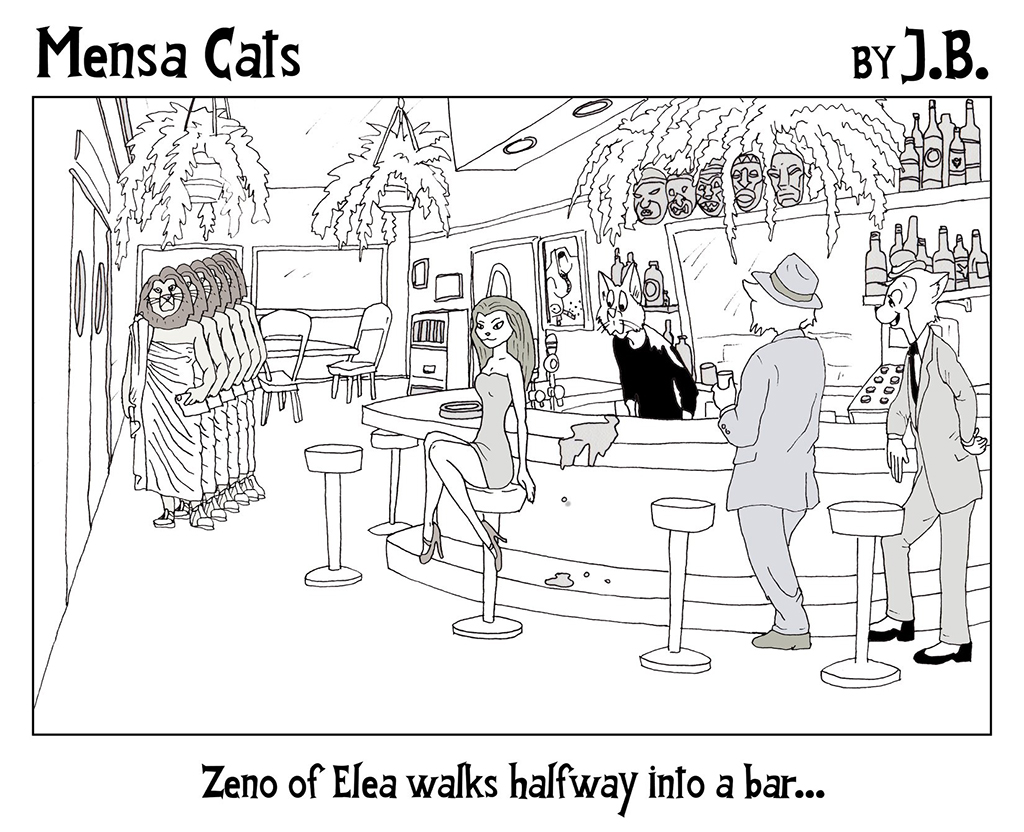# Mensa Cat Monday: Zeno’s ParadoxesWe bring you a brand new Mensa Cats cartoon by J.B. (that’s Jason Berry of Vacuum Tree Head).  This one expresses not one but two of Zeno’s famous paradoxes in a single frame.

The first is the better-known Dichotomy Paradox, from which the punchline derives.  In order to walk into the bar, Zeno (or any of the other patrons) must first walk halfway; and to travel from the halfway point, he must travel half of that distance, and then half of that distance, and so on.  It seems that this process of walking halfway would continue forever and one would not arrive and one’s destination, yet we know in reality that we do.

The second paradox is the Arrow Paradox.  Consider the frame frozen in time, as indicated by the liquid in mid spill behind the young lady cat, or the multiple instances of Zeno along his path.  At any given moment, there is no motion. Zeno’s position does change between moment, but there is an infinite number of other frames, each with a fixed position.  As copies/frames of Zeno get closer and closer together, the change in time between them gets infinitesimally small.  Yet the process 0f adding up these infinite frames with zero motion in each results in Zeno walking halfway into the bar.

The two paradoxes (and the third, commonly referred to as “Achilles and the tortoise”) are closely related.  The first deals with infinitesimal subdivisions of space, while the second deals with infinitesimal subdivisions of time.  The key is that while any infinitesimal quantity is smaller than any quantity we can express, it is always still greater than zero.  And adding up an infinite number of infinitesimal quantities can sometimes yield a finite number.

Take the halving process in the Dichotomy paradox.  Zeno moves half this distance, then half of that, and so on.  This can be expressed as an infinite sum.As one gets closer to infinity, the sum gets closer and closer to one.  More formally, we can say that as we approach infinity, the sum goes to 1.  So an infinite number of subdivisions still reaches our goal of the full distance.

This process of applying operations to an infinite number of infinitesimal subdivisions is the principal behind calculus, and a marvelous thing to behold once one gets used to it.  The mathematics does not necessarily answer the metaphysical questions raised by these ancient paradoxes, but it is what most interests us at CatSynth.  It’s been a while since we last shared the joy and beauty of mathematics on these pages, but it is high time we resume the practice.

# Calculus for Cats and Prime Number Theorem

<I was looking for a quick way to combine cats and mathematics this morning, and came across the book Calculus for Cats.

This is a book for people about to take calculus, and for survivors of calculus who still wonder what it was all about. It gently explains the basic concepts and vocabulary without making the reader ever do a single problem.

Basically, the book draws (quite literally) an analogy between the fluid motion of cats at play (or in pursuit of “prey”) and the concepts and techniques of calculus, which focuses on continuous functions.

We at CatSynth remember calculus fondly as a mathematical pursuit. But number theory is more my thing. Calculus primary concerns itself with continuous functions of real and complex numbers, while number theory deals with discrete entities, like integers. But in mathematics, all things are interconnected. For example, we demonstrated the connection between the gamma function, pi and factorials, combining continuous and discrete concepts.

Consider the function π(x), the prime-counting function. It's a bit unfortunate they chose the symbol π, but it is what it is. Basically, this function counts the number of primes less than or equal to a particular number. For example π(20) would be all the prime numbers less than 20: 2, 3, 5, 7, 11, 13, 17 and 19. So π(20) = 8.

So to calculate π(1000) would one have to literally count all the prime numbers less than 1000, including figuring out which numbers are prime? And what about π(1000000)? Unfortunately, the answer is yes. But there are good ways to approximate the number of primes, using the results of the Prime Number Theorem. Those interested in the formal theorem are encouraged to follow the link, but we will skip ahead to one of the interesting results. One of basic functions to come out of calculus is the natural logarithm ln(x), whose base is the famous constant e. If you don't know about it, go look it up. Otherwise, the rest of this article will not make much sense. One can use ln(x) to build more complicated functions in calculus, one of which is the offset logarithmic integral, or Li(x):This is one of those functions, like the gamma function, that cannot be expressed without the use of calculus. Turns out, however, that it is a good approximately for π(x), which is very much a discrete concept and quite distant from the continuous motions involved in calculus. The prime number theorem provides the connection.